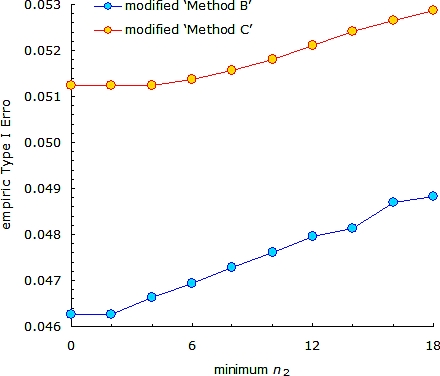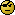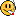Helmut
★★★Vienna, Austria,
2016-12-30 01:22

Posting: # 16908
Views: 4,852

## Impact of minimum stage 2 sample size on the Type I Error [Two-Stage / GS Designs]

Dear all,

on a recent occasion… We know that the minimum n2 = 2 as required in the Q&A document is meaningless. Either a study stops in the first stage or it continues with at least two subjects anyway.However, do not go further unless you know what you are doing. If you require a minimum stage 2 sample size all studies where a smaller sample size would already be sufficient to demonstrate BE with the target power are now forced to this size. Higher sample size ⇒ more degrees of freedom ⇒ narrower CI ⇒ higher probability to pass BE.
In other words, the TIE will also increase and one would have to use a lower adjusted α.

To the right an example what would happen if one modifies Potvin’s Methods B and C at the location (n1 12, CV 20%) of the maximum TIE and naïvely applies the ‘natural constant’ α 0.0294.

Not a very good idea. Own simulations are mandatory in order to find a suitable α!

Cheers,
Helmut SchützThe quality of responses received is directly proportional to the quality of the question asked. 🚮
Science Quotes
ElMaestro
★★★

Belgium?,
2016-12-30 12:12

@ Helmut
Posting: # 16910
Views: 4,160

## Impact of minimum stage 2 sample size on the Type I Error

Hi Helmut,

I am sure you are right but I can't follow you, I mean can't readily understand what question you tried to answer.
So let me ask the forbidden question: "Can you reformulate?"

» Higher sample size ⇒ more degrees of freedom ⇒ narrower CI ⇒ higher probability to pass BE.
» In other words, the TIE will also increase and one would have to use a lower adjusted α.

This is one thing I did not get. Does that logic also work when we simulate true GMR 0.8 or 1.25 for type I error? I find it hard to convince myself.

Somehow I guess regulators just wanted to say that inclusion of a single subject in stage 2 would not be ok. They are right and that is not rocket science.

This week's list of things I absolutely detest: Corona virus, the which function in R, WIA-WIA interfaces for scanning under Windows 10, the Bee Gees, the smell of my fridge.

Best regards,
ElMaestro
Helmut
★★★Vienna, Austria,
2016-12-30 14:01

@ ElMaestro
Posting: # 16911
Views: 4,233

## Impact of minimum stage 2 sample size on the TIE: example

Hi ElMaestro,

» So let me ask the forbidden question: "Can you reformulate?"
»
» » Higher sample size ⇒ more degrees of freedom ⇒ narrower CI ⇒ higher probability to pass BE.
» » In other words, the TIE will also increase and one would have to use a lower adjusted α.
»
» This is one thing I did not get.

I’ll give two examples. Both at the location (n1 12, CV 20%) of the maximum TIE.
Simulating for power (at 0.95):
1. No lower limit of n2
library(Power2Stage) power.2stage(method="B", alpha=rep(0.0294, 2), CV=0.2,              n1=12, GMR=0.95, targetpower=0.8, min.n2=0) TSD with 2x2 crossover Method B: alpha (s1/s2) = 0.0294 0.0294 Target power in power monitoring and sample size est. = 0.8 Power calculation via non-central t approx. CV1 and GMR = 0.95 in sample size est. used No futility criterion BE acceptance range = 0.8 ... 1.25 CV = 0.2; n(stage 1) = 12; GMR= 0.95 1e+05 sims at theta0 = 0.95 (p(BE)='power'). p(BE)    = 0.84174 p(BE) s1 = 0.41333 Studies in stage 2 = 56.34% Distribution of n(total) - mean (range) = 20.6 (12 ... 82) - percentiles  5% 50% 95%  12  18  40

2. Lower limit of n2 = 1.5 × n1
power.2stage(method="B", alpha=rep(0.0294, 2), CV=0.2,              n1=12, GMR=0.95, targetpower=0.8, min.n2=18) TSD with 2x2 crossover Method B: alpha (s1/s2) = 0.0294 0.0294 Target power in power monitoring and sample size est. = 0.8 Power calculation via non-central t approx. CV1 and GMR = 0.95 in sample size est. used No futility criterion Minimum sample size in stage 2 = 18 BE acceptance range = 0.8 ... 1.25 CV = 0.2; n(stage 1) = 12; GMR= 0.95 1e+05 sims at theta0 = 0.95 (p(BE)='power'). p(BE)    = 0.91564 p(BE) s1 = 0.41333 Studies in stage 2 = 56.34% Distribution of n(total) - mean (range) = 23.5 (12 ... 82) - percentiles  5% 50% 95%  12  30  40
If we require that the minimum sample size in the second stage = 1.5 × n1, naturally the same percent of studies will proceed to the second stage. However, the expected total sample sizes will be larger (E[N] 23.5 vs. 20.6, median 30 vs. 18). The sponsor gains power (91.6% vs. 84.2%).

» Does that logic also work when we simulate true GMR 0.8 or 1.25 for type I error? I find it hard to convince myself.

Yes, it does – and this was my point. This time simulating for the TIE (at 1.25):
1. No lower limit of n2
library(Power2Stage) power.2stage(method="B", alpha=rep(0.0294, 2), CV=0.2,              n1=12, GMR=0.95, targetpower=0.8, min.n2=0, theta0=1.25) TSD with 2x2 crossover Method B: alpha (s1/s2) = 0.0294 0.0294 Target power in power monitoring and sample size est. = 0.8 Power calculation via non-central t approx. CV1 and GMR = 0.95 in sample size est. used No futility criterion BE acceptance range = 0.8 ... 1.25 CV = 0.2; n(stage 1) = 12; GMR= 0.95 1e+06 sims at theta0 = 1.25 (p(BE)='alpha'). p(BE)    = 0.046262 p(BE) s1 = 0.028849 Studies in stage 2 = 87.86% Distribution of n(total) - mean (range) = 23.1 (12 ... 98) - percentiles  5% 50% 95%  12  22  40

2. Lower limit of n2 = 1.5 × n1
power.2stage(method="B", alpha=rep(0.0294, 2), CV=0.2,              n1=12, GMR=0.95, targetpower=0.8, min.n2=18, theta0=1.25) TSD with 2x2 crossover Method B: alpha (s1/s2) = 0.0294 0.0294 Target power in power monitoring and sample size est. = 0.8 Power calculation via non-central t approx. CV1 and GMR = 0.95 in sample size est. used No futility criterion Minimum sample size in stage 2 = 18 BE acceptance range = 0.8 ... 1.25 CV = 0.2; n(stage 1) = 12; GMR= 0.95 1e+06 sims at theta0 = 1.25 (p(BE)='alpha'). p(BE)    = 0.048816 p(BE) s1 = 0.028849 Studies in stage 2 = 87.86% Distribution of n(total) - mean (range) = 29.2 (12 ... 98) - percentiles  5% 50% 95%  12  30  40
The Type I Error increases from 0.046262 (no minimum n2) to 0.048816 (minimum n2 = 1.5 × n1). No problem with ‘Method B’ but gets nasty with ‘Method C’ (see the plot in my OP).

» Somehow I guess regulators just wanted to say that inclusion of a single subject in stage 2 would not be ok. They are right and that is not rocket science.

I think not to perform the second stage with one subject is a no-brainer. I guess that two was a compromise. AFAIK, Alfredo suggested 12 subjects to the BSWP.*

• García-Arieta A, Gordon J. Bioequivalence Requirements in the European Union: Critical Discussion. AAPS J. 2012;14(4):738–48. doi:10.1208/s12248-012-9382-1

Cheers,
Helmut SchützThe quality of responses received is directly proportional to the quality of the question asked. 🚮
Science Quotes
ElMaestro
★★★

Belgium?,
2016-12-30 17:19

@ Helmut
Posting: # 16913
Views: 4,099

## Impact of minimum stage 2 sample size on the TIE: example

Hi Helmut,

I am still lost, I must confess. Perhaps it is because I am not using the power2Stage package at all.

» I’ll give two examples. Both at the location (n1 12, CV 20%) of the maximum TIE.
» Simulating for power (at 0.95):
1. No lower limit of n2
» library(Power2Stage)
» power.2stage(method="B", alpha=rep(0.0294, 2), CV=0.2,
»              n1=12, GMR=0.95, targetpower=0.8, min.n2=0)

Should this not be min.n2=2 ? Or is it the "same difference"??

Do you think you have it in your heart to explain in slow motion to a dimwit like me who read your posts quite a few times which point you are trying to prove or investigating?

Otherwise I am afraid I will need to question you next time we meet f2f. And that might not be in the distant futureThis week's list of things I absolutely detest: Corona virus, the which function in R, WIA-WIA interfaces for scanning under Windows 10, the Bee Gees, the smell of my fridge.

Best regards,
ElMaestro
Helmut
★★★Vienna, Austria,
2016-12-30 18:00

@ ElMaestro
Posting: # 16914
Views: 4,144

## Impact of minimum stage 2 sample size on the TIE: example

Hi ElMaestro,

» » Simulating for power (at 0.95):
1. No lower limit of n2
» » library(Power2Stage)
» » power.2stage(method="B", alpha=rep(0.0294, 2), CV=0.2,
» »              n1=12, GMR=0.95, targetpower=0.8, min.n2=0)

» Should this not be min.n2=2 ?

Nope. This is the original Potvin ‘Method B’. There is no minimum n2 in the paper, right? However, the functions in Power2Stage are constructed in such a way that (in cross-over TSDs) any estimated sample size has to be an even number. If one states min.n2=1 it will automatically rounded up to 2. Same goes with sampleN.TOST(). Hence, to state min.n2=2 is a waste of time (see also the footnote to this post).

» Do you think you have it in your heart to explain in slow motion to a dimwit like me who read your posts quite a few times which point you are trying to prove or investigating?

I’ll try. Without a minimum n2 what would happen in a study which – following the conditions of the framework – could proceed to the second stage? n2 could be any even number. Say we had n1 24 and estimate the total sample size N (for stage 1 CV, assumed GMR and target power) with 30. Hence, n2 6.
If we mandate n2 = max(n2 = 1.5n1, Nn1) we have to perform the second stage in 36 instead of 6. In the pooled analysis we will have 60 subjects instead of 30. Much higher power (nice for wealthy sponsors) but not so nice if we look at the TIE. Since the final size is twice as large, the chance to pass BE (at 1.25) will be larger as well. Even if we keep everything equal the DFs come into play. Therefore, the ‘original’ adjusted α might not sufficiently control the TIE – and we would need a lower one.
That’s pure reasoning (wetware).» Otherwise I am afraid I will need to question you next time we meet f2f. And that might not be in the distant futureReally? Great!

Cheers,
Helmut SchützThe quality of responses received is directly proportional to the quality of the question asked. 🚮
Science Quotes
ElMaestro
★★★

Belgium?,
2016-12-30 18:50

@ Helmut
Posting: # 16915
Views: 4,040

## Impact of minimum stage 2 sample size on the TIE: example

Ah, got it, thanks Helmut,

» (...a bunch of blah blah blah...)
» That’s pure reasoning (wetware).I think you are saying that:
• the alpha2 that works for n2,min=2 or 0 or 1 or whatever is not necessarily the alpha2 that works for n2,min=1.5*n1, all other factors being equal.
That is correct. I don't think it is something I personally can deduce logically by looking at the algo or equations, but it is a correct statement, I believe, based on simulations.
It is tempting to say power increases with sample size, and since type I error is a kind of power, this is the logic behind the observation. I think the issue is somewhat more complex than just that. These two-stage thingies are funny objects that defy all kinds of logic.

Does it change anything though?? I mean you and I both argued in the past that universally functional alpha's do not exist, so whenever someone makes a smart/clever/sophisticated/dumb/intelligent/braindead amendment to Potvin B or C etc, then simulations should always be undertaken to make sure the type I error is not compromised.

This week's list of things I absolutely detest: Corona virus, the which function in R, WIA-WIA interfaces for scanning under Windows 10, the Bee Gees, the smell of my fridge.

Best regards,
ElMaestro
Helmut
★★★Vienna, Austria,
2016-12-30 19:00

@ ElMaestro
Posting: # 16916
Views: 4,083

## Bingo!

Hi ElMaestro,

now you got it!

» – the alpha2 that works for n2,min=2 or 0 or 1 or whatever is not necessarily the alpha2 that works for n2,min=1.5*n1, all other factors being equal.

Yes.

» It is tempting to say power increases with sample size, and since type I error is a kind of power, this is the logic behind the observation.

Yes.

» I think the issue is somewhat more complex than just that. These two-stage thingies are funny objects that defy all kinds of logic.

Maybe and yes.

» Does it change anything though??

For me, no.

» I mean you and I both argued in the past that universally functional alpha's do not exist, so whenever someone makes a smart/clever/sophisticated/dumb/intelligent/braindead amendment to Potvin B or C etc, then simulations should always be undertaken to make sure the type I error is not compromised.

Exactly. To quote Jones and Kenward.*

[…] before using any of the methods […], their operating characteristics should be evaluated for a range of values of n1, CV and true ratio of means that are of interest, in order to decide if the Type I error rate is controlled, the power is adequate and the potential maximum total sample size is not too great.

(my emphases)

• Jones B, Kenward MG. Design and analysis of cross-over trials. Boca Raton: Chapman & Hall/CRC; 2014. p. 365–80.

Cheers,
Helmut SchützThe quality of responses received is directly proportional to the quality of the question asked. 🚮
Science QuotesIng. Helmut Schütz